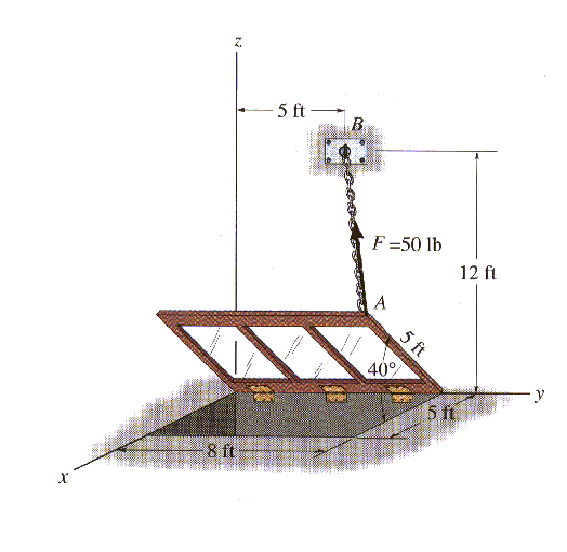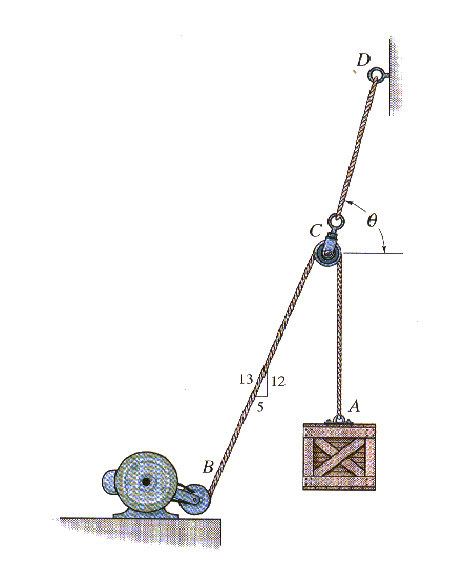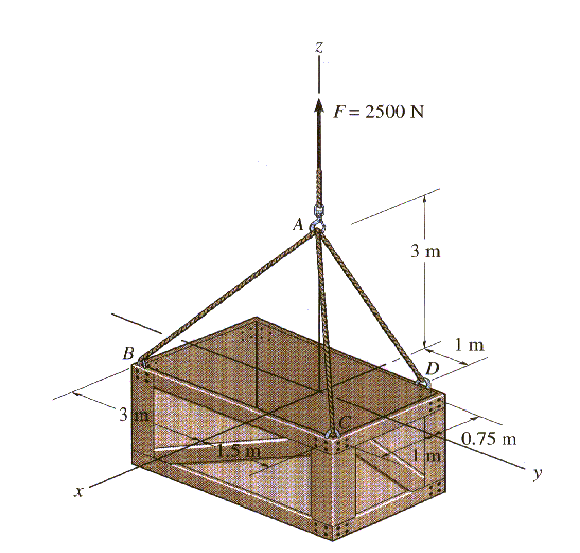Statics-Sample Exam I

Note to the students: The following is a typical "Exam I" for statics. It should give you an idea of the difficulty of the exam. Try to do the exam in 50 minutes without the aid of your book or your notes, only using your one page of equations (no solutions to problems). For each problem, first layout the procedure to obtain the desired solution, then complete the problem by doing all the details. Don't forget to draw free-body diagrams for the equilibrium problems, and to present your result in three significant digits with proper units. If the figures are not clear, you can find them in the book.

Problem 1: The window is held open by chain AB. Determine the length of the chain, and express the 50-lb force acting at A along the chain as a Cartesian vector. Determine its coordinate direction angles.Problem 2: The cords BCA and CD can each support a maximum load of 100-lb. Determine the maximum weight of the crate that can be hoisted at constant velocity, and the angle q for equilibrium.Problem 3: The 2500-N crate is to be hoisted with constant velocity from the hold of a ship using the cable arrangement shown. Determine the tension in each of the three cables for equilibrium.